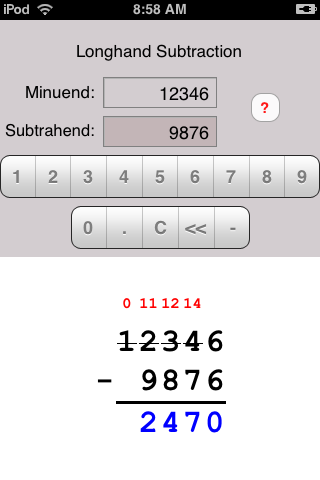Make Math Work
iPhone® and iPod Touch® Longhand Subtraction
 Home

Welcome to Longhand Subtraction
Longhand Subtraction is a no-nonsense easy-to-use application available on the Apple iTunes Store. With Longhand Subtraction you can subtract two numbers and see the problem solved in columnar form. If borrowing is necessary the borrowing is displayed.

Quick Note
Longhand Subtraction works with all iOS devices running iOS 2.1 or greater. However, if your device uses iOS 4.3 or greater, please consider purchasing Longhand Math instead of Longhand Subtraction. Here's why:
Longhand Math...
• Does addition, subtraction, multiplication and division
• It's Longhand Addition, Longhand Subtraction, Longhand Multiplication and Longhand Division rolled into one product.
• Purchasing the four products above would cost almost \$4.00
• It looks better and is optimized for iPhone 5
Take A Look At Longhand Math

Longhand Subtraction ScreensHow To Use Longhand Subtraction
Select either the minuend or the subtrahend by touching the text entry box for the particular item. Touch the number buttons to enter numbers into each selected item. Touch the minus (-) button to calculate the difference between the minuend and subtrahend.

Minuend and Subtrahend
Touch either text entry box to select it.

0-9
Enter digits into the minuend and subtrahend.

Decimal Point (.)
Enter a decimal point into a number.

<<
Clear the rightmost digit in the minuend or subtrahend.

C
Completely clear the selected number item.

-
Subtract the digits (minuend minus the subtrahend) and display the result.

?
Switch to the help screen.

Even More Help
If your question has not been answered you are welcome to email us at support@MakeMathWork.com. Please include:
1. The name of this application: Longhand Subtraction.
2. A detailed description of the problem.
3. The exact steps to reproduce the problem.
4. A screenshot if possible.